﻿ E r r 库存例证. 插画 包括有 设备, 附庸风雅, 概念, 计算机国际庞克, 背包, 比赛, 受控 - 146959361

# E r. 设备, 附庸风雅.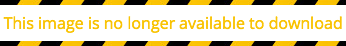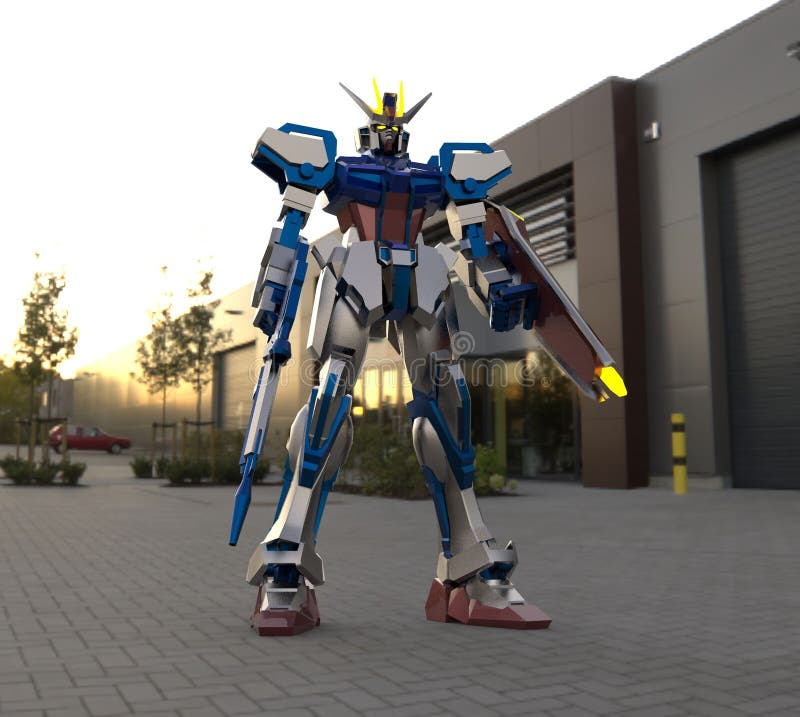E r r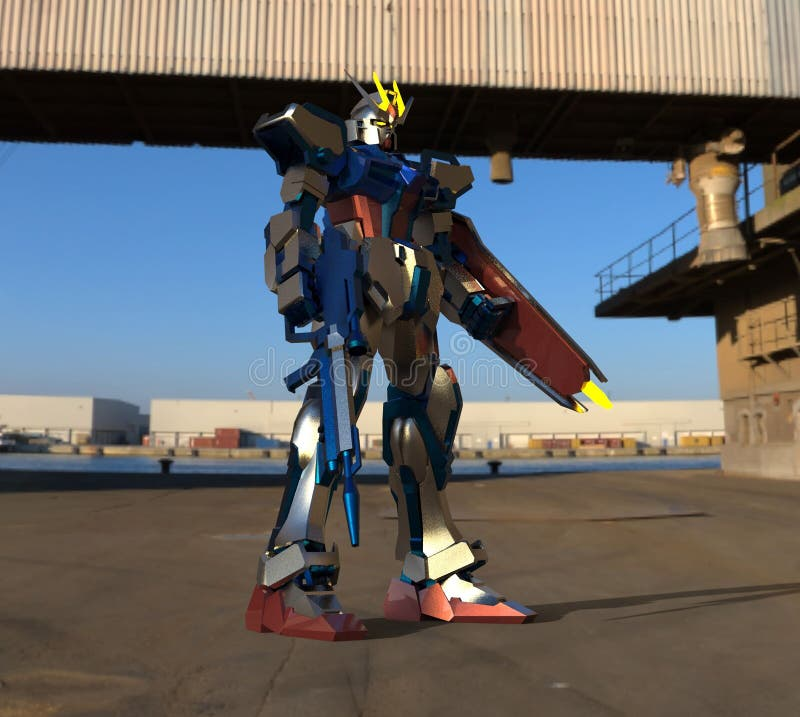E r r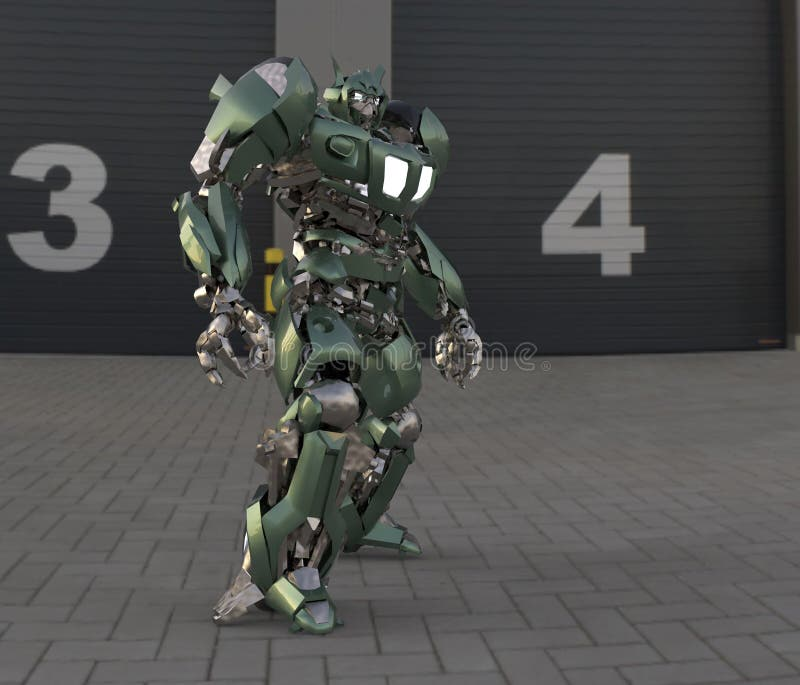E r r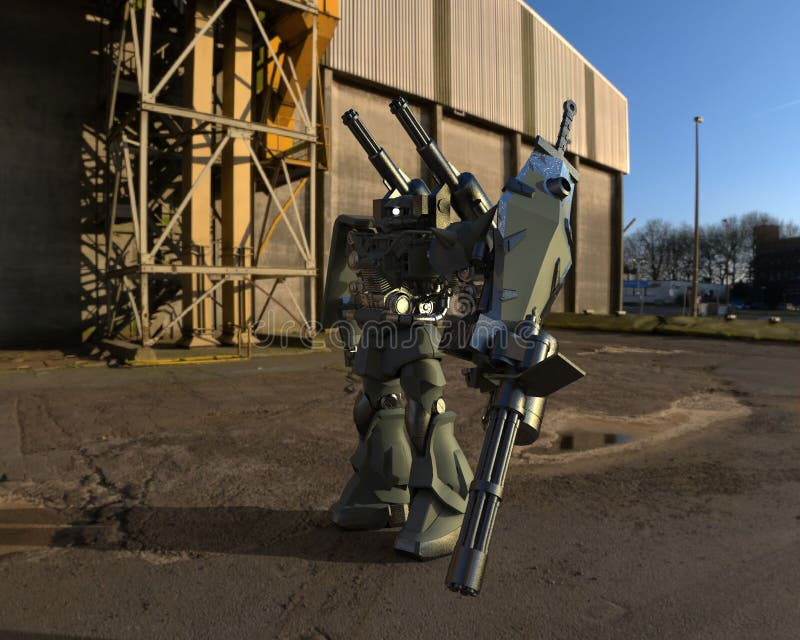E r r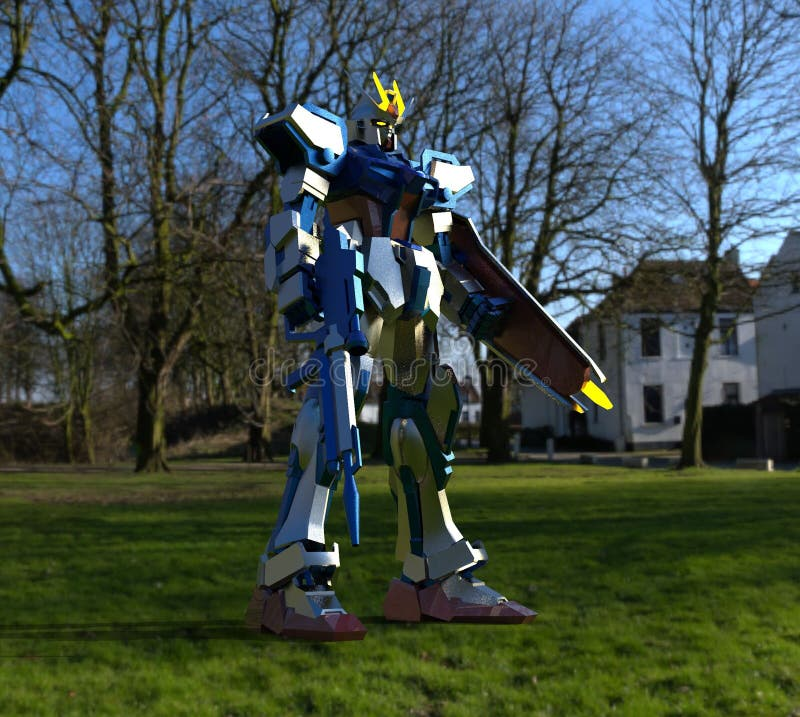E r r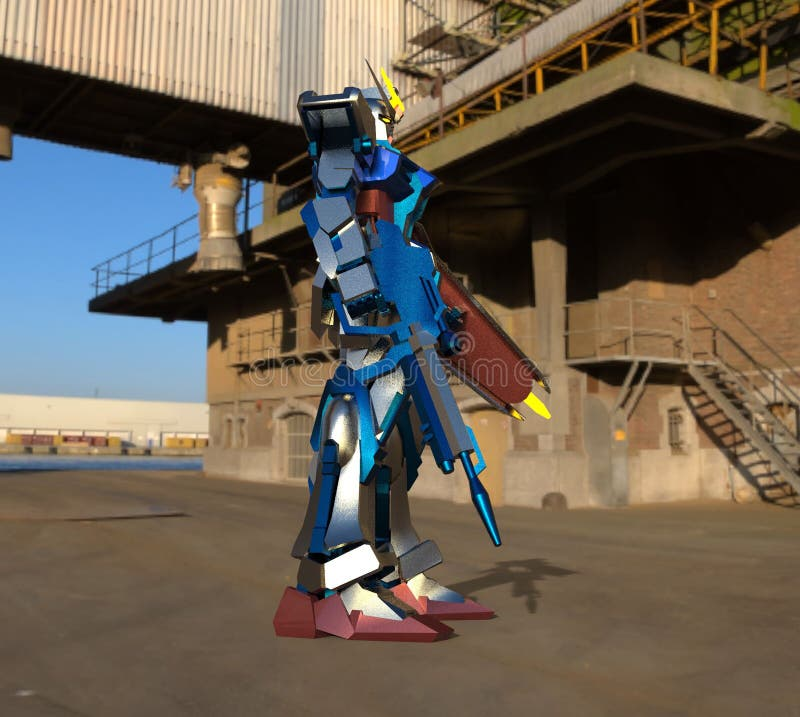E r r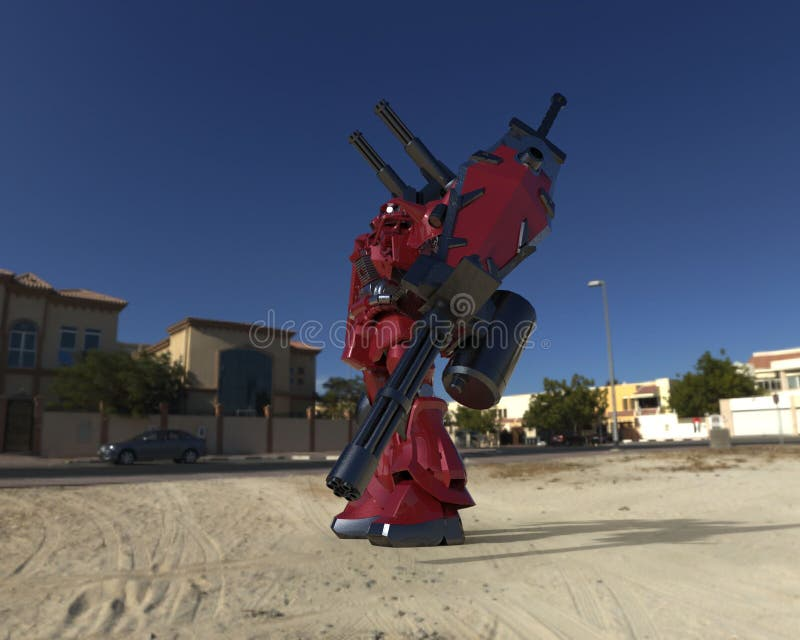E r r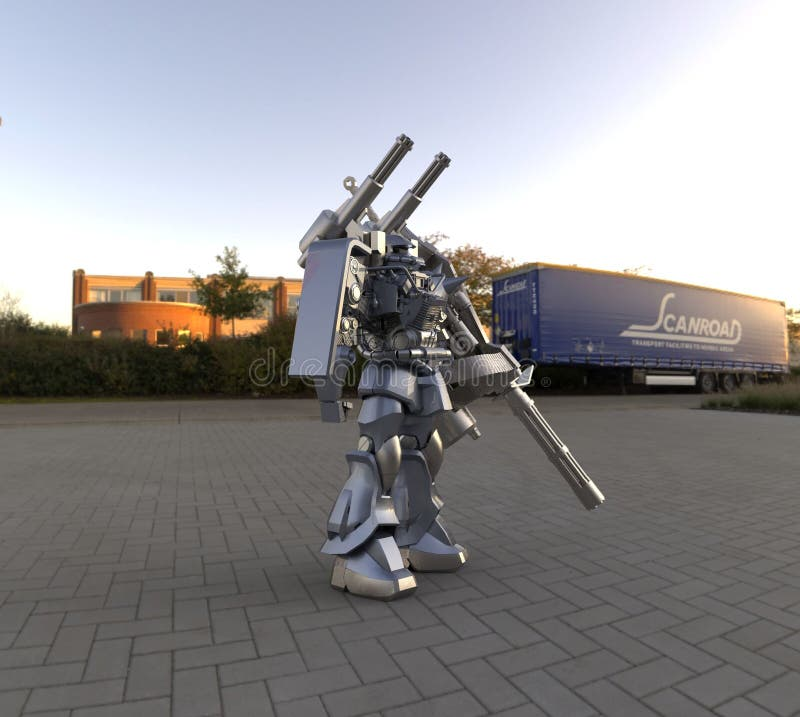E r r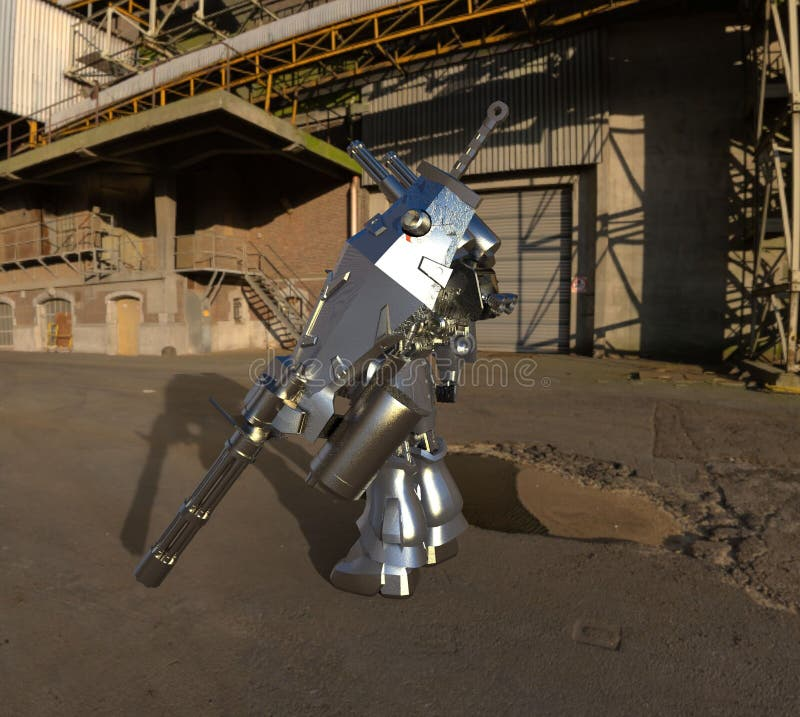E r r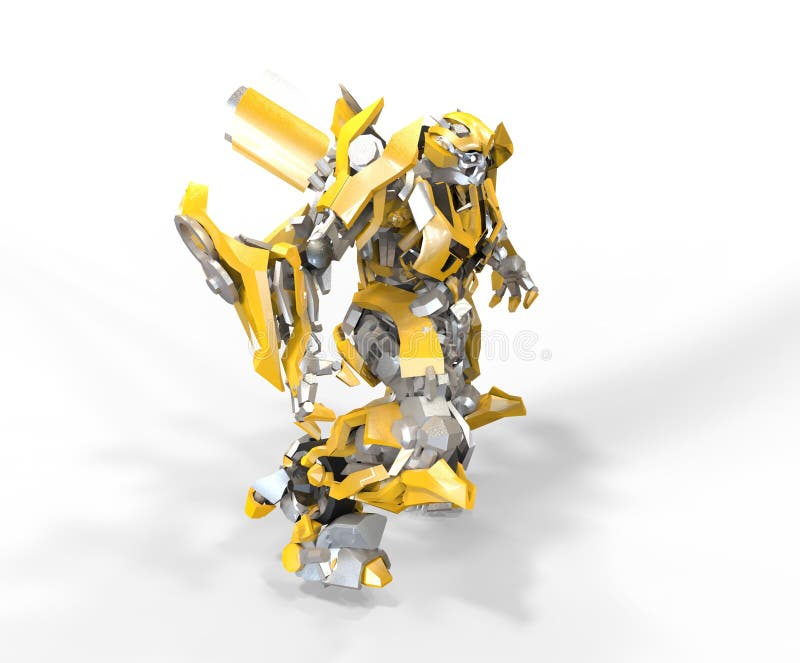E r r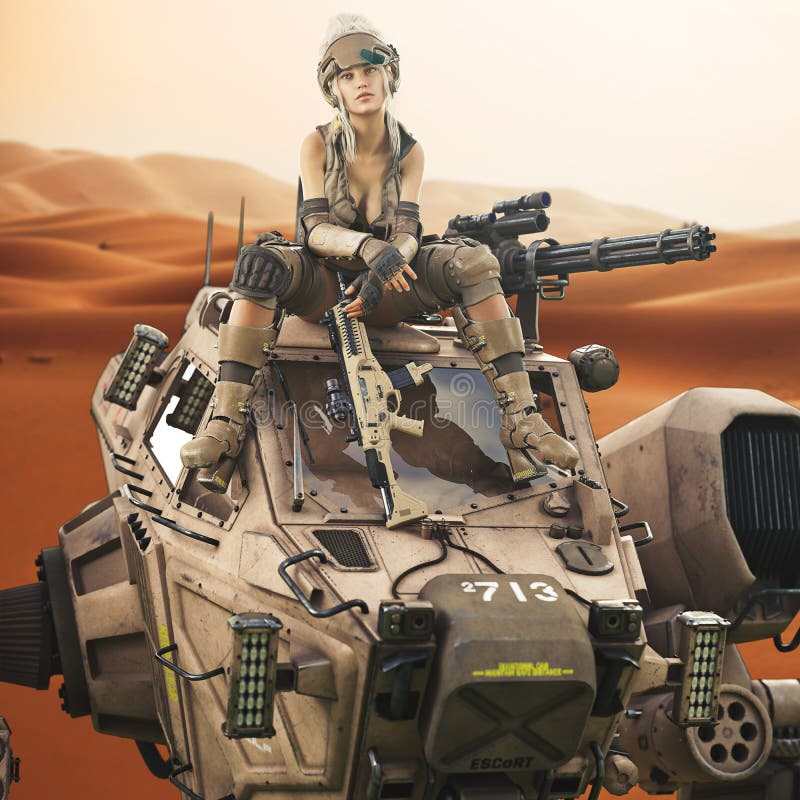坐在她的被驾驶的机械机器人机器顶部的未来派女兵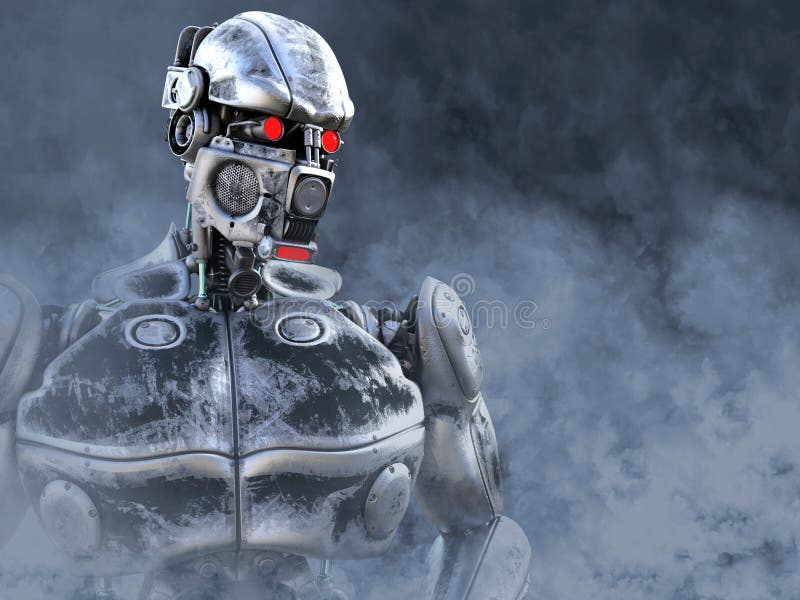3D一位未来派机械战士的翻译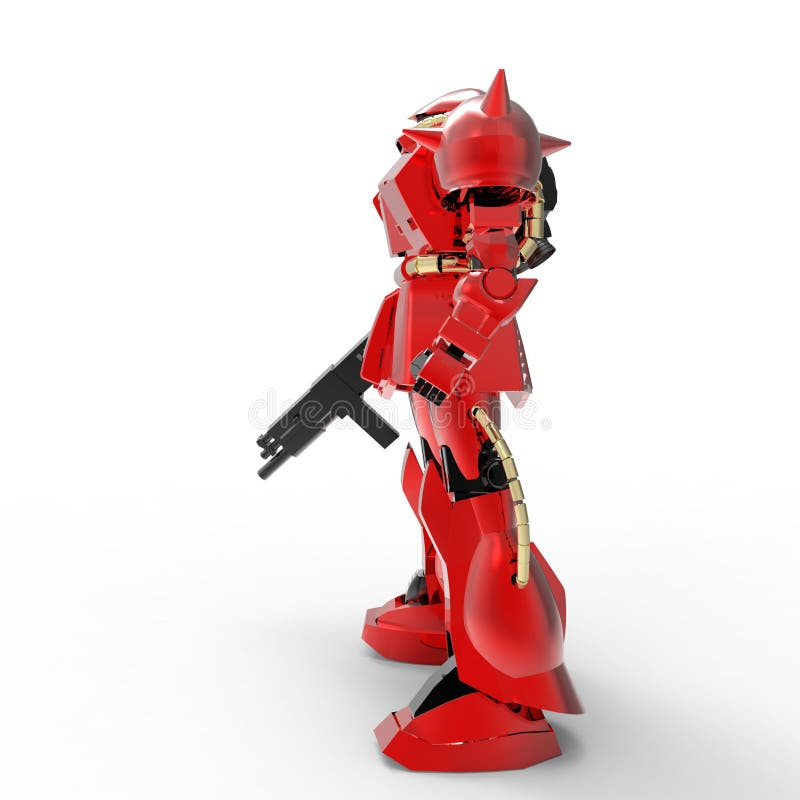E r 机械受控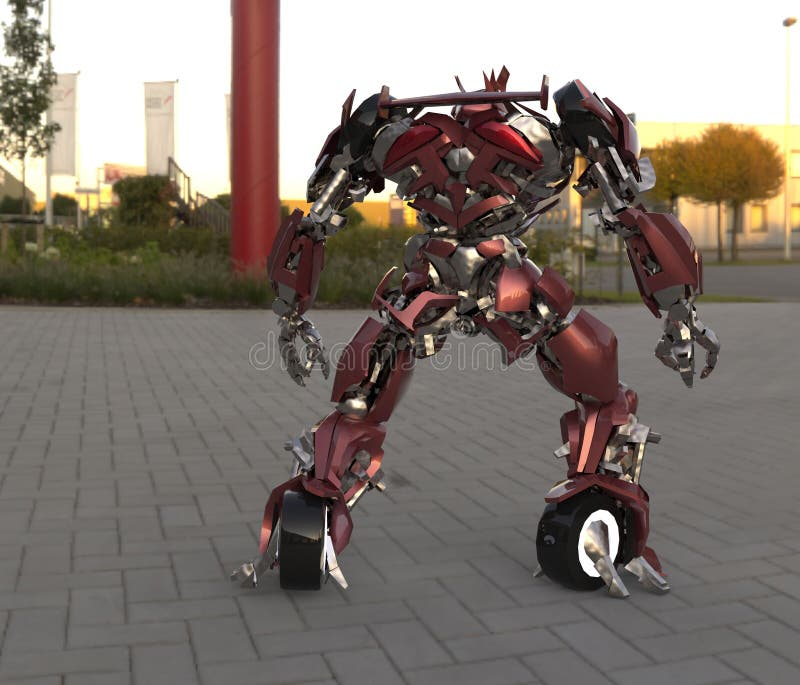在风景背景的科学幻想小说机械战士身分 r ??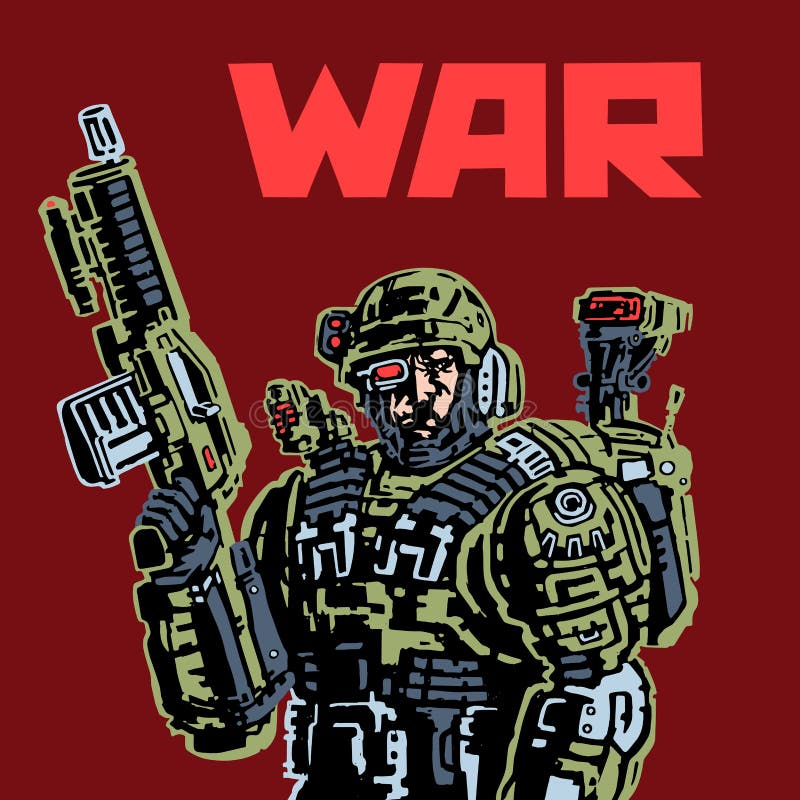未来的靠机械装置维持生命的人战士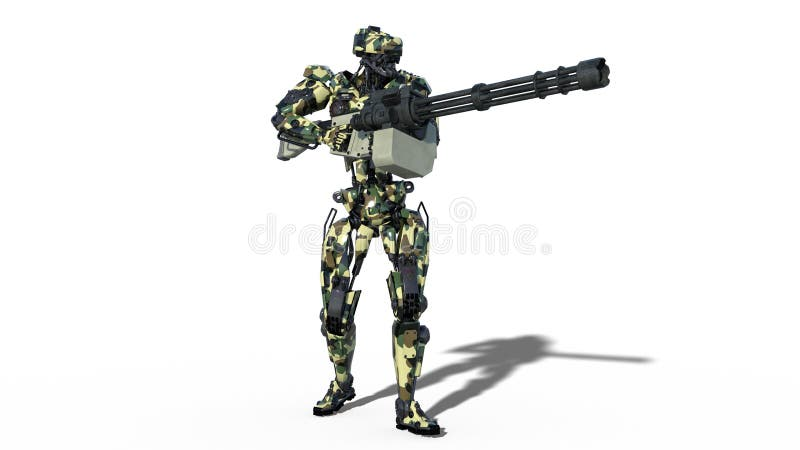军队机器人，武力靠机械装置维持生命的人，在白色背景， 3D的军用机器人战士射击机枪回报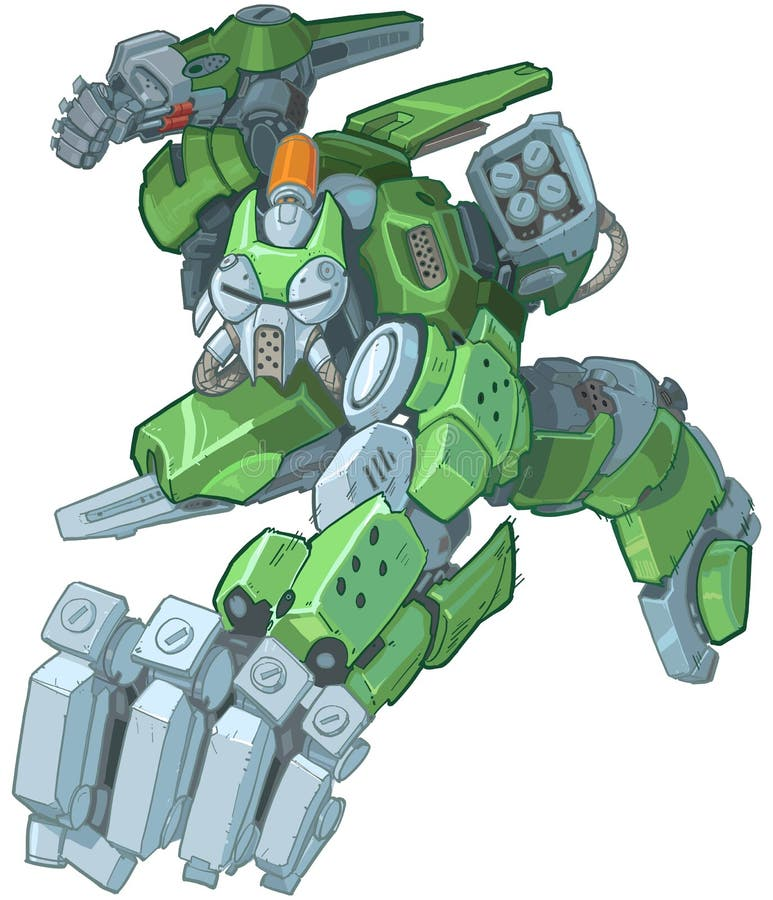有人的特点的绿色动画片战士机器人猛击的例证科学幻想小说军用巨型争斗机器 有人的特点的机器人在启示乡下 极糟的社会，科幻，机械和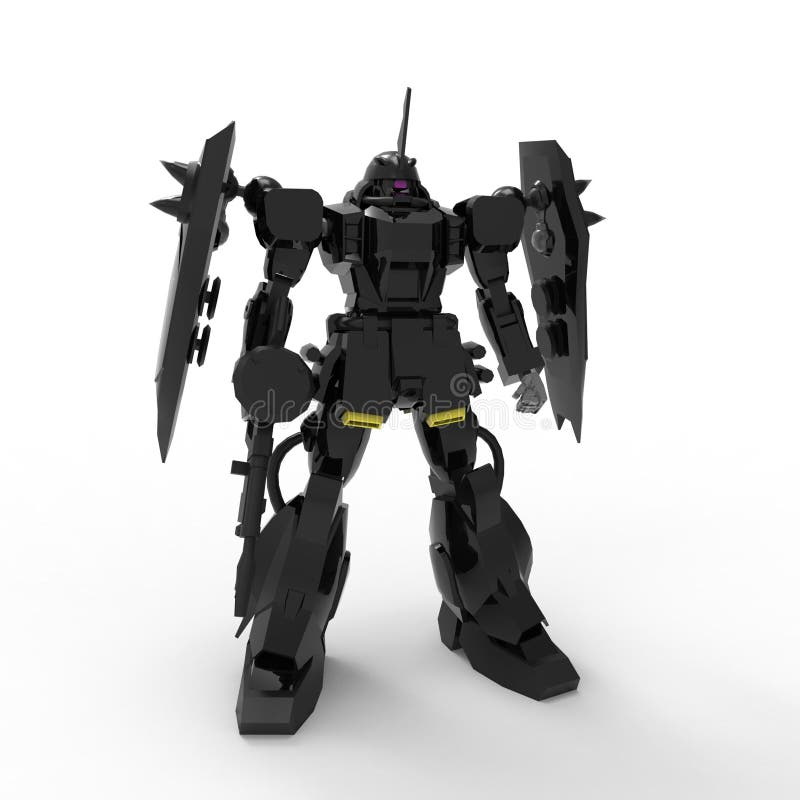E r 机械受控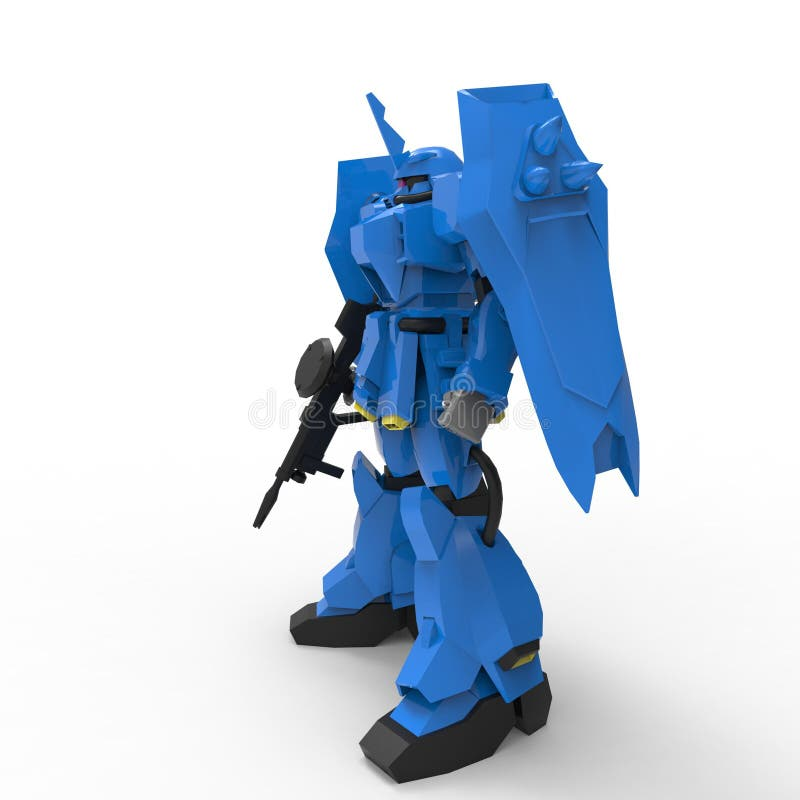E r 机械受控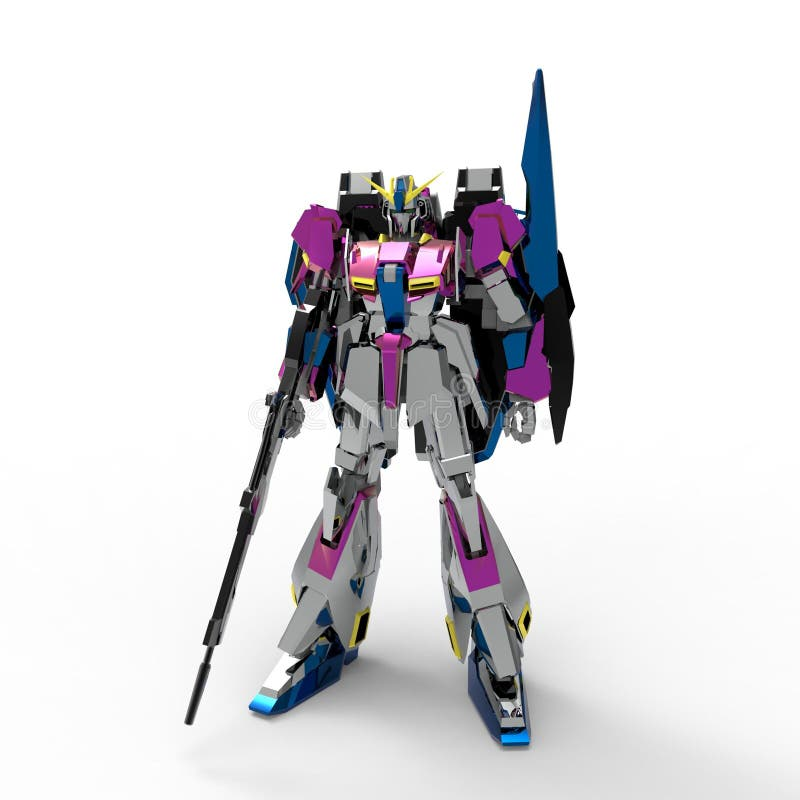E r 受控制的机械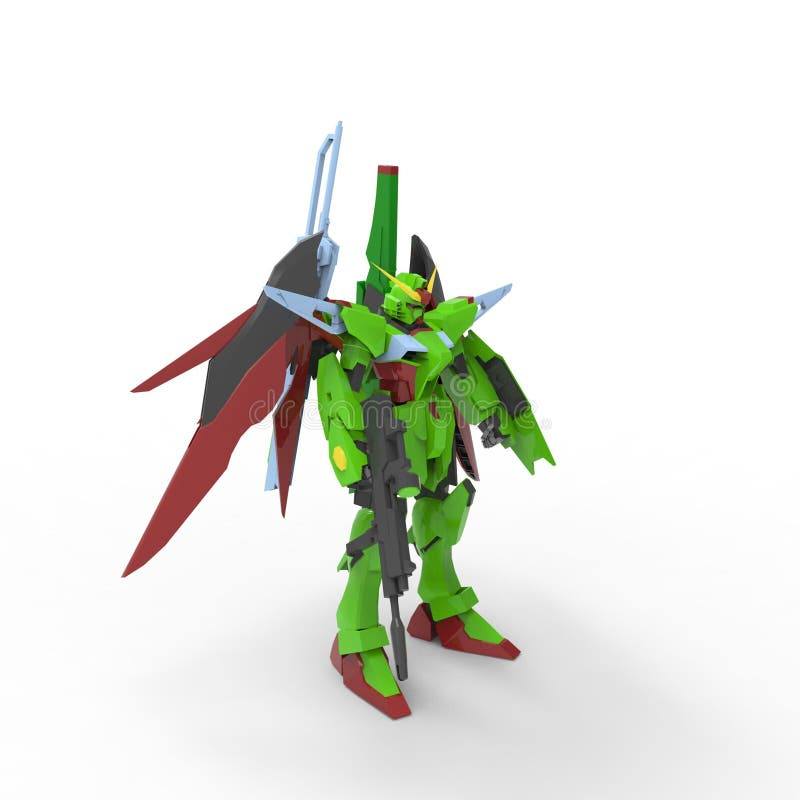E r 机械受控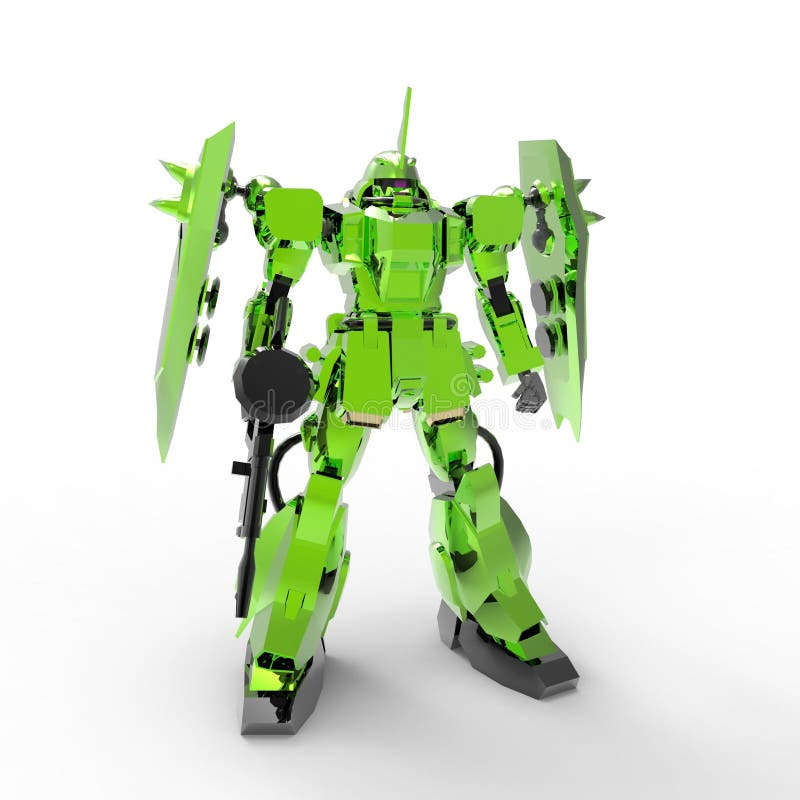E r 机械受控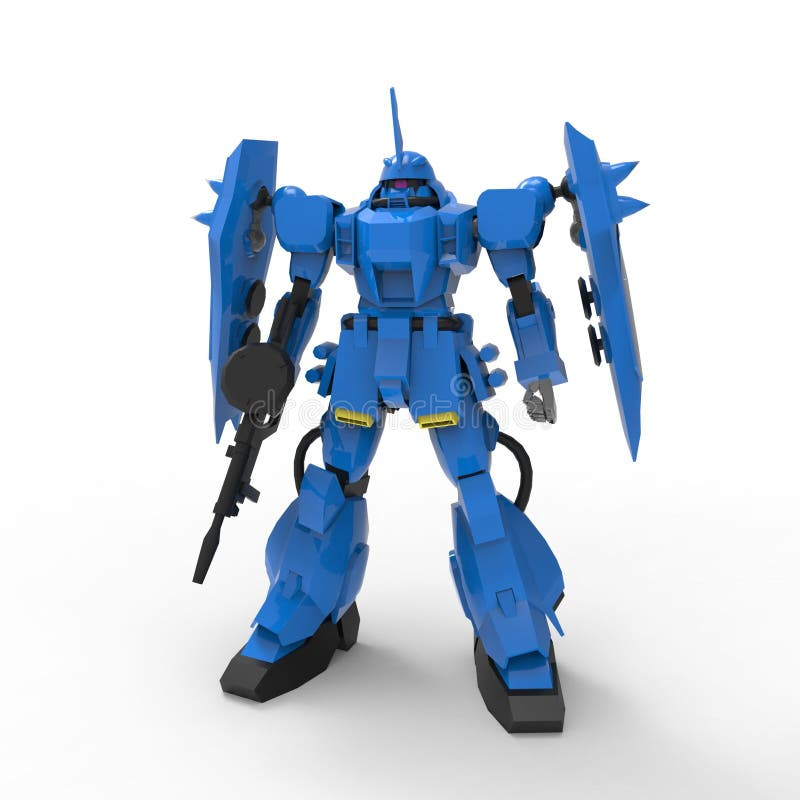E r 机械受控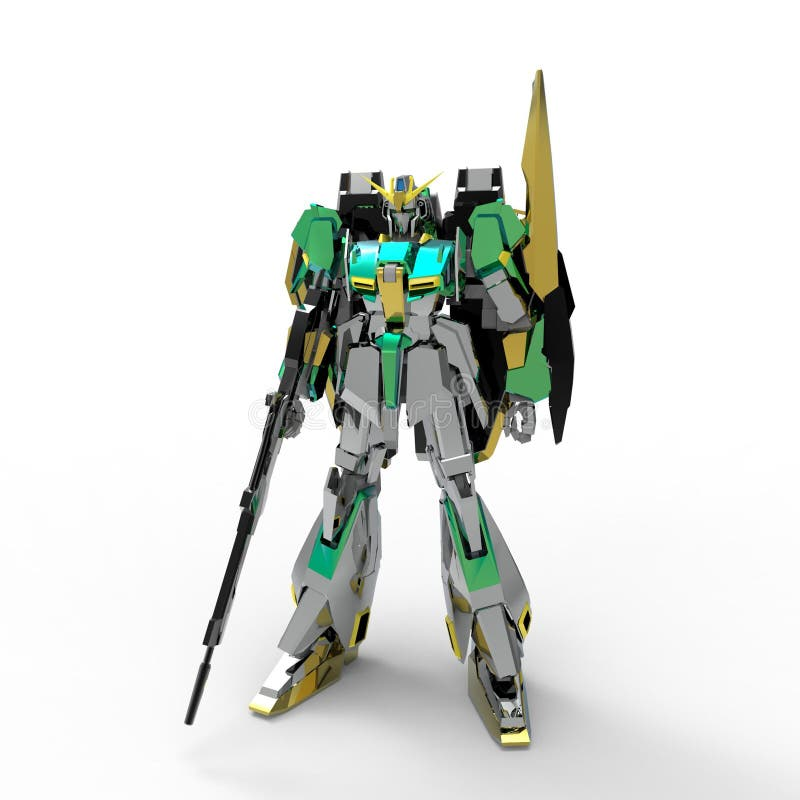E r 受控制的机械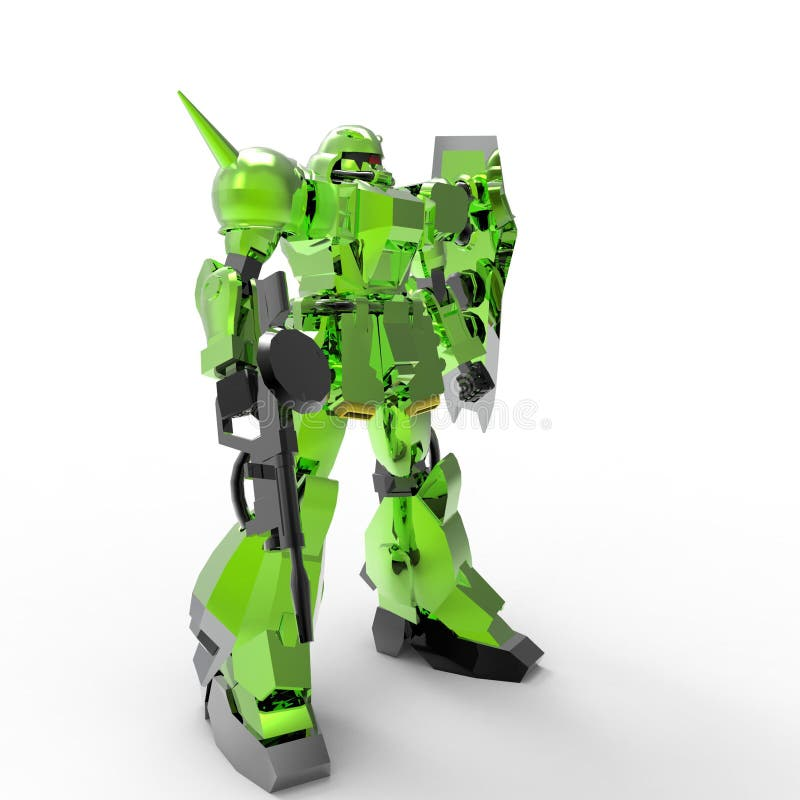E r 受控制的机械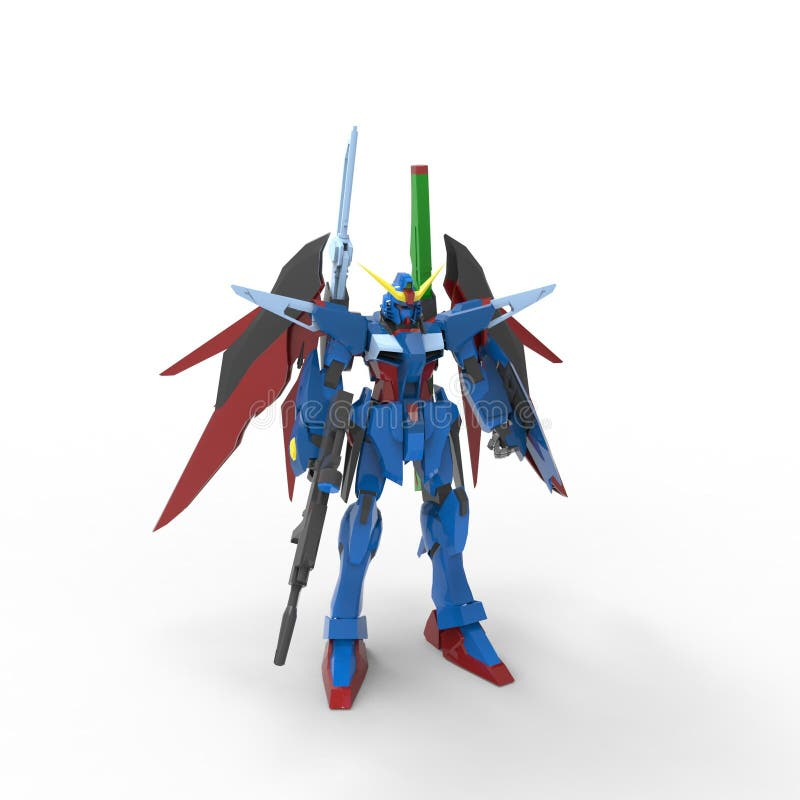E r 机械受控